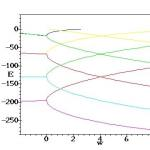## Exactly solvable problems in quantum mechanicsSupervisor Email address levai.geza[~S+P-Á-M]atomki.mta.hu Research area Quantum physics Participants in the research work Publications
Abstract

Quantum systems are usually described in terms of the Schrödinger equation that contains a potential accounting for the interactions. The solutions are usually obtained by numerical techniques but sometimes they can be written in exact mathematical form. The importance of exactly solvable problems lies in the fact that they can be used to develop and test numerical techniques. Furthermore, they give insight into the fundamental aspects of quantum mechanics, e.g. the symmetries of the system.

Description

The exact description of quantum mechanical potential problems is usually performed by  transforming the Schrödinger equation into the second-order differential equation of some special function of mathematical physics. Depending on the choice of this function and on the variable transformation, various classes of exactly solvable potentials can be obtained. The most widely discussed potentials belong to the six-parameter Natanzon class, in which case the solutions of the Schrödinger equation are obtained in terms of a single hypergeometric or confluent hypergeometric function. The most widely known exactly solvable potentials (harmonic oscillator, Coulomb, Pöschl-Teller etc.) are two- or three-parameter subclasses of the Natanzon class.

Supersymmetric quantum mechanics (SUSYQM) is another standard tool of analysing exactly solvable potentials. This method can be used to generate new exactly solvable potentials from known ones such that the two potentials are isospectral, except perhaps for their ground states.

There are several ways to obtain exactly solvable potentials beyond the Natanzon class. One is considering further special functions of mathematical physics (e.g. the Bessel function, Heun functions etc.), while another one is considering the linear combination of several special functions of the same type in the solutions.

The variable transformation method, as well as the SUSY transformations can also be connected with group theoretical techniques, which allow for the discussion of various symmetry concepts related to the potentials.

PT-symmetric quantum mechanics introduced in 1998 is a non-hermitian formulation of quantum mechanics with complex potentials. The Hamiltonian of these systems is invariant under the simultaneous space (P) and time (T) inversion, and has real energy eigenvalues as long as PT symmetry is unbroken. The standard methods have been adapted to this theory too, leading to a large number of exactly solvable PT-symmetric potentials.

Bender, CM ; Lévai, G: Exactly Solvable PT-Symmetric Models
In: Tateo, Roberto; Lévai, Géza; Kuzhel, Sergii; Jones, Hugh F; Hook, Daniel W; Fring, Andreas; Dunning, Clare; Dorey, Patrick E; Bender, Carl M:
​PT Symmetry in Quantum and Classical Physics
London, UK / World Scientific (Europe), (2019) pp. 221-260. , 40 p.